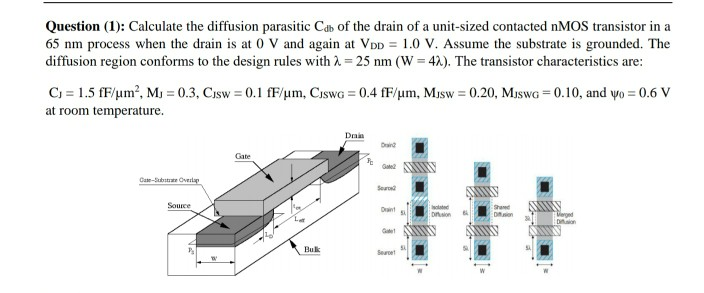# Question Solved1 AnswerQuestion (1): Calculate the diffusion parasitic Cdb of the drain of a unit-sized contacted nMOS transistor in a 65 nm process when the drain is at O V and again at Vpp = 1.0 V. Assume the substrate is grounded. The diffusion region conforms to the design rules with 2 = 25 nm (W = 42). The transistor characteristics are: C) = 1.5 fF/um?, M, = 0.3, Cosw = 0.1 fF/um, Ciswa = 0.4 fF/um, Musw = 0.20, Mjswg = 0.10, and yo = 0.6 V at room temperature. Dei Gate Po Cate-State Overlap MS Source D. org Dion AN Bulk SeurX8PQNF The Asker · Electrical EngineeringTranscribed Image Text: Question (1): Calculate the diffusion parasitic Cdb of the drain of a unit-sized contacted nMOS transistor in a 65 nm process when the drain is at O V and again at Vpp = 1.0 V. Assume the substrate is grounded. The diffusion region conforms to the design rules with 2 = 25 nm (W = 42). The transistor characteristics are: C) = 1.5 fF/um?, M, = 0.3, Cosw = 0.1 fF/um, Ciswa = 0.4 fF/um, Musw = 0.20, Mjswg = 0.10, and yo = 0.6 V at room temperature. Dei Gate Po Cate-State Overlap MS Source D. org Dion AN Bulk Seur
More
Transcribed Image Text: Question (1): Calculate the diffusion parasitic Cdb of the drain of a unit-sized contacted nMOS transistor in a 65 nm process when the drain is at O V and again at Vpp = 1.0 V. Assume the substrate is grounded. The diffusion region conforms to the design rules with 2 = 25 nm (W = 42). The transistor characteristics are: C) = 1.5 fF/um?, M, = 0.3, Cosw = 0.1 fF/um, Ciswa = 0.4 fF/um, Musw = 0.20, Mjswg = 0.10, and yo = 0.6 V at room temperature. Dei Gate Po Cate-State Overlap MS Source D. org Dion AN Bulk Seur